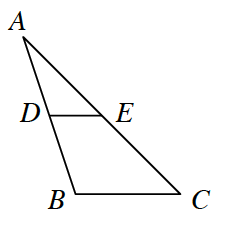### Home > INT2 > Chapter 11 > Lesson 11.1.1 > Problem11-8

11-8.In the diagram at right, $\overline{DE}$ is a midsegment of $ΔABC$. If the area of $ΔABC$ is $96$ square units, what is the area of $ΔADE$? Explain how you know.

$ΔADE\simΔABC$

What is the linear scale factor?
What is the ratio of the areas?

$24$ square units.